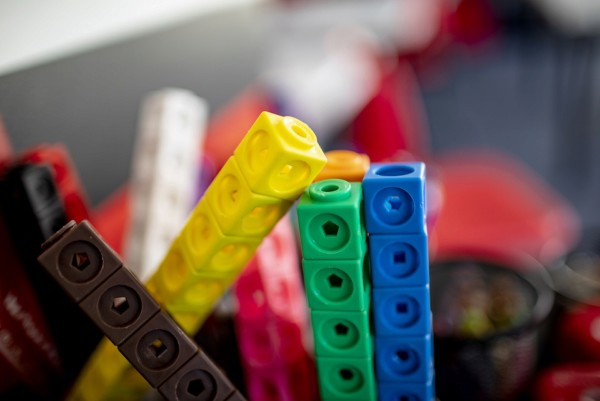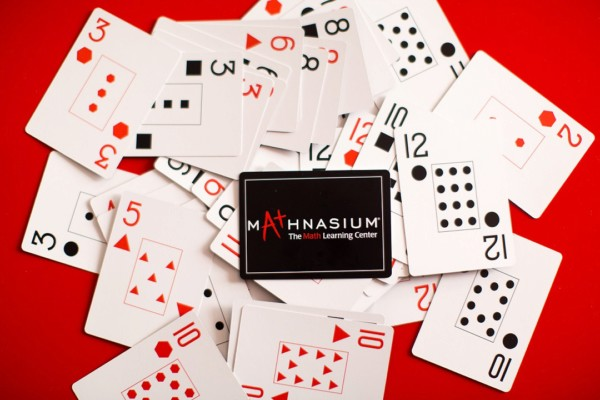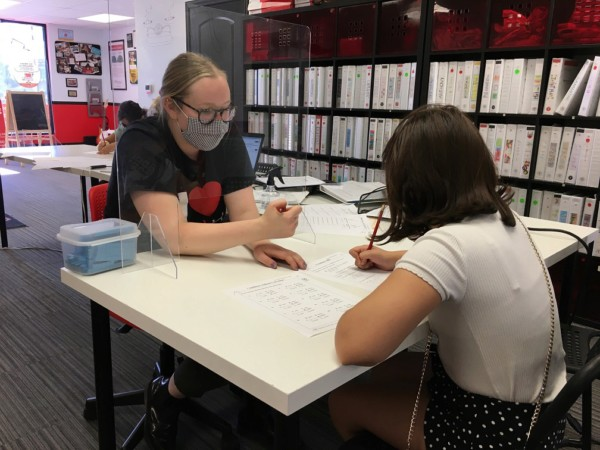877-601-6284
Get Started by Finding a Local Center

# How Math Knowledge Builds on Itself

Aug 31, 2020 | Location Eagle Rock

### By Chloe, Instructor

If you’ve been reading this blog for a while, you’ve seen me talk about how math knowledge builds on itself, constantly growing and expanding, and requiring a solid foundation and understanding before new concepts can be introduced. Other subjects, like history, don’t work this way. While having an understanding of the years leading up to the American Revolution adds context and understanding to learn about the event, you don’t have to know anything about those years to grasp the concept.

Math is completely different. If you don’t understand the basics, it will be impossible to further your learning until you’ve spent time practicing and mastering those skills. In this article, I’m going to write a bit more about why that is, and explain how vital it is that skills are learned to mastery while kids are still young. (If you aren't sure whether your child has the basics down, Mathnasium offers a free math skills assessment that will help you to know where they are at.)

1. Complements of 10Early on in math learning, while you’re still learning the basics (i.e. single-digit numbers), you’ll also learn that specific pairs of those numbers add together to make 10: 1 and 9, 2 and 8, and so on. This may seem like a simple enough concept, but if you don’t have an intuitive understanding of which numbers are complements of one another, math gets a lot harder when you start working with bigger numbers. Figuring out how far it is from 37 to 91 becomes a much greater challenge if you don’t remember that 7 and 3 make 10 (or in this case, that 37 and 3 make 40). Learning these values and complements feels like such a minor thing, but since all of our math exists in a base-10 numbering system, it’s one of the most important things to know.
2. Addition and SubtractionAdding and subtracting small numbers is a simple enough skill to learn. This many plus this many more is how many altogether? Or, if I start with this many, and this many are taken away, how many are left? Since kids are used to playing with small objects, the concept can usually be grasped easily. As soon as you move on to the next stage, adding and subtracting larger numbers, learning can get bogged down in the set-up rather than the solution. If you don’t have the skill to envision the problem you’re solving, setting it up, and finding the answer can be extremely difficult. 437 beads minus 189 beads isn’t a problem that can be easily solved on your fingers, and counting it out would take a long time. Learning the basics of adding and subtracting makes this next jump in learning a lot easier.
3. Multiplication and DivisionOnce you’ve understood the concept of adding and subtracting, you can introduce the idea of multiplication and division. Multiplication is really just repeated addition a specific number of times. 3x4 is just 3 + 3 + 3 + 3 (Four 3s). This particular technique isn’t always taught, and when it is, it isn’t always learned, but the ability to visualize a multiplication problem as an addition problem helps tremendously when you get into larger numbers. While memorizing multiplication tables is necessary and useful, understanding that (7x8) is the same thing as (7x7 + 7) can help you solve problems even if you’ve forgotten one or two of the facts you memorized, and can even help you find answers that are well beyond what children are taught to memorize (which usually stops at 12x12).
4. Place ValueMath makes a big leap when you introduce the concept of place value. Suddenly, you aren’t just adding or multiplying complements of 10 or 100, but can be combining and breaking apart numbers in the thousands or tens of thousands. If you haven’t mastered your basic skills, a problem like (4,614,621 – 703,018) might look like a foreign language (and that’s because it is—math is a language like any other, with rules, grammar, and syntax). “Lining up the place values and borrowing” is a set of rules you can learn to help solve the problem, but only as long as numbers are still being used in the problems. Once you’ve moved into algebra, if you don’t know how to visualize the problem outside of the rules you’ve learned, you’ll have trouble learning anything new.
5. Greater Than/Less ThanRecognizing that one number is bigger or smaller than another requires an understanding of place value and other basic number facts, like complements of ten and the order of numbers. Understanding which symbol to use is a separate skill and one that becomes even more important when you move into higher math like algebra. (9 > 7) is fairly clear, but (x < 3y) means something entirely different. If you haven’t learned what it means early on, it certainly won’t make more sense when you advance.

This is just the beginning, but hopefully I was able to convince you that math is a skill that just keeps building and expanding on itself, much like the use of language itself. Knowing how part of it works helps you to understand how other pieces fit, and fully understanding it makes future learning a lot easier.

If you notice that your child/student is falling behind in one or more of these skills, it’s more important to get them the extra help now, before the concepts begin to feel so far out of reach that they stop trying to learn them altogether.

##### Locations near
Could not find Center, try again